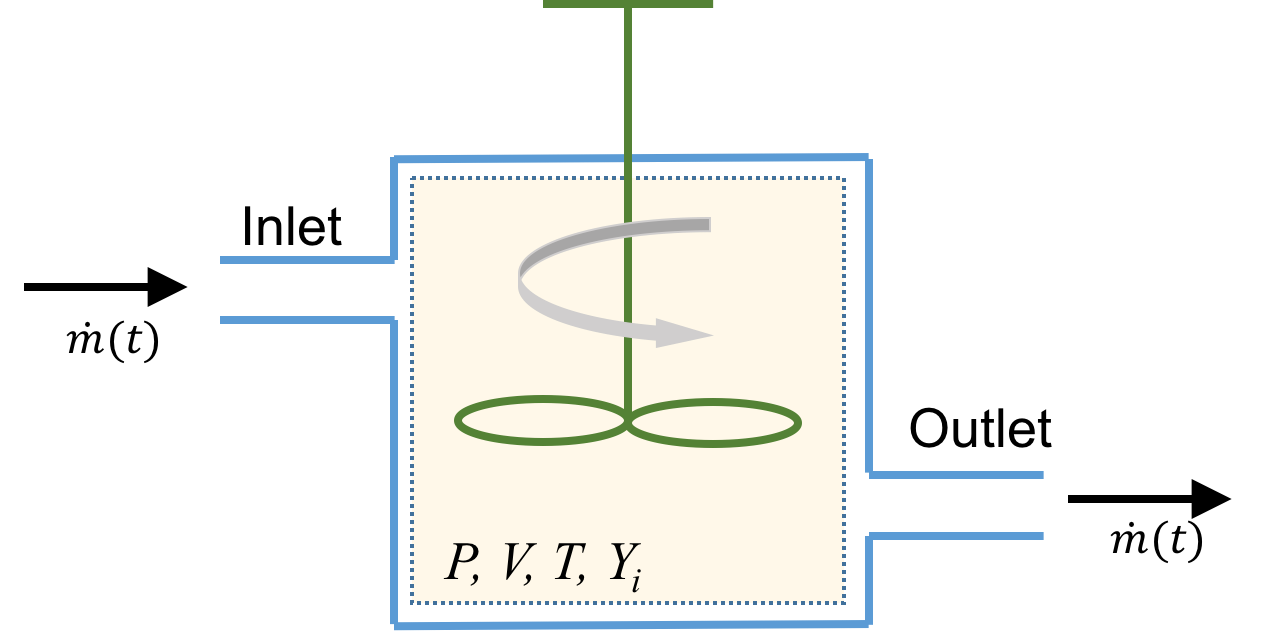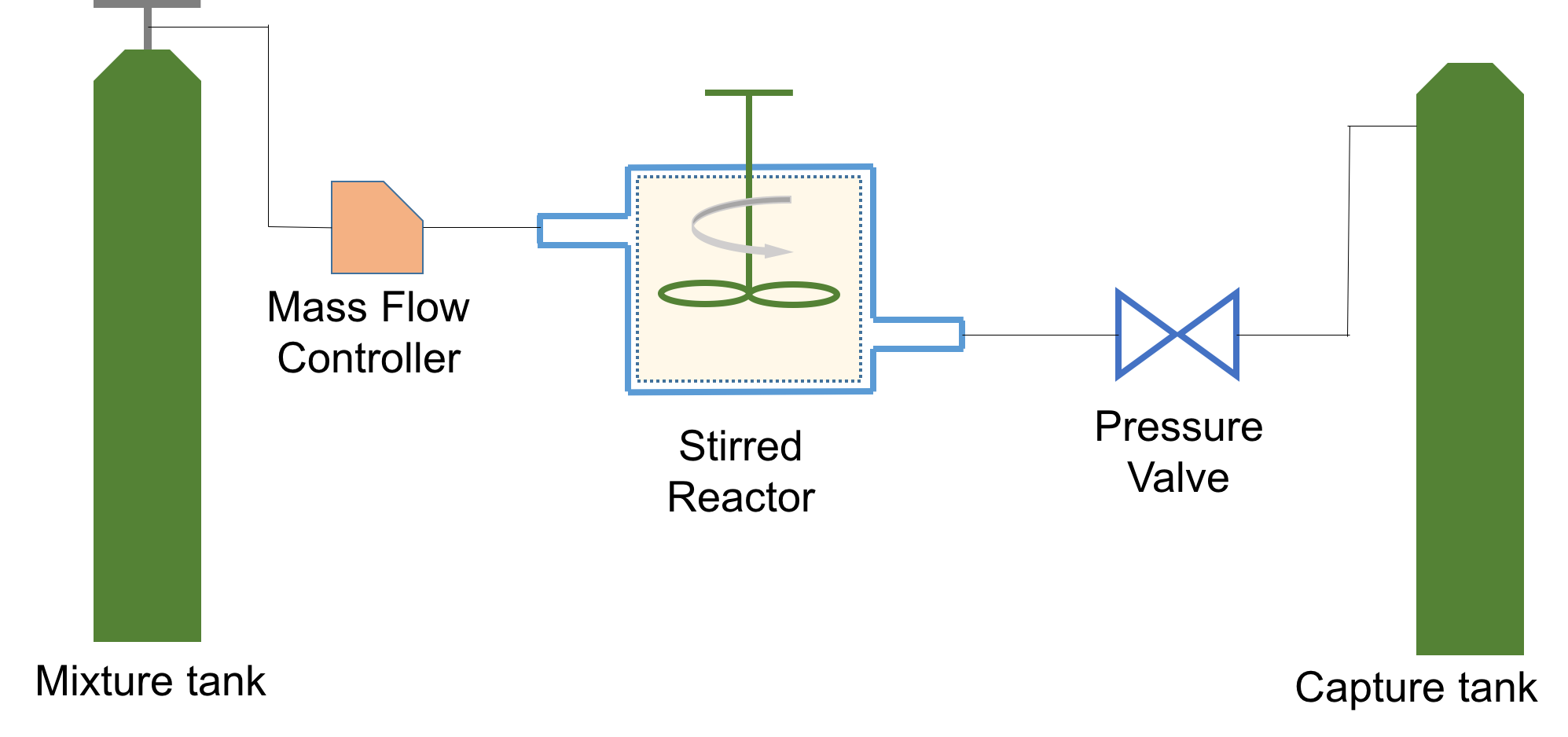# stirred_reactor.ipynb (Source)

Data files used in this example: galway.yaml, zhangExpData.csv

## Continuous Reactor¶

In this example we will illustrate how Cantera can be used to simulate a Continuously Stirred Tank Reactor (CSTR), also interchangeably referred to as a Perfectly Stirred Reactor or a Well Stirred Reactor, a Jet Stirred Reactor or a Longwell Reactor (there may well be more "aliases").

A cartoon of such a reactor is shown below:### Simulation of a CSTR/PSR/WSR¶

As the figure illustrates, this is an open system (unlike a Batch Reactor which is isolated). P, V and T are the reactor's pressure, volume and temperature respectively. The mass flow rate at which reactants come in is the same as that of the products which exit; and these stay in the reactor for a characteristic time $\tau$, called the residence time. This is a key quantity in sizing the reactor and is defined as follows:

\begin{equation*} \tau = \frac{m}{\dot{m}} \end{equation*}

where $m$ is the mass of the gas

In :
import pandas as pd
import time
import cantera as ct

print(f"Running Cantera version: {ct.__version__}")

Running Cantera version: 2.6.0a4


### Import modules and set plotting defaults¶

In :
%matplotlib inline
%config InlineBackend.figure_formats = ["svg"]
import matplotlib.pyplot as plt

plt.style.use("ggplot")
plt.style.use("seaborn-pastel")

plt.rcParams["axes.labelsize"] = 18
plt.rcParams["xtick.labelsize"] = 14
plt.rcParams["ytick.labelsize"] = 14
plt.rcParams["figure.autolayout"] = True
plt.rcParams["figure.dpi"] = 120


### Define the gas¶

In this example, we will work with $nC_{7}H_{16}$/$O_{2}$/$He$ mixtures, for which experimental data can be found in the paper by Zhang et al.. We will use the same mechanism reported in the paper. It consists of 1268 species and 5336 reactions

In :
gas = ct.Solution("../data/galway.yaml")

CanteraWarning: NasaPoly2::validate:
For species OHV, discontinuity in h/RT detected at Tmid = 1000.0
Value computed using low-temperature polynomial:  53.62056162666667
Value computed using high-temperature polynomial: 53.5841554314

CanteraWarning: NasaPoly2::validate:
For species CHV, discontinuity in h/RT detected at Tmid = 1000.0
Value computed using low-temperature polynomial:  107.5046684
Value computed using high-temperature polynomial: 107.34847808033334



### Define initial conditions¶

#### Inlet conditions for the gas and reactor parameters¶

In :
# Inlet gas conditions
reactor_temperature = 925  # Kelvin
reactor_pressure = 1.046138 * ct.one_atm  # in atm. This equals 1.06 bars
inlet_concentrations = {"NC7H16": 0.005, "O2": 0.0275, "HE": 0.9675}
gas.TPX = reactor_temperature, reactor_pressure, inlet_concentrations

# Reactor parameters
residence_time = 2  # s
reactor_volume = 30.5 * (1e-2) ** 3  # m3


#### Simulation parameters¶

In :
# Simulation termination criterion
max_simulation_time = 50  # seconds


### Reactor arrangement¶

We showed a cartoon of the reactor in the first figure in this notebook; but to actually simulate that, we need a few peripherals. A mass-flow controller upstream of the stirred reactor will allow us to flow gases in, and in-turn, a "reservoir" which simulates a gas tank is required to supply gases to the mass flow controller. Downstream of the reactor, we install a pressure regulator which allows the reactor pressure to stay within. Downstream of the regulator we will need another reservoir which acts like a "sink" or capture tank to capture all exhaust gases (even our simulations are environmentally friendly !). This arrangment is illustrated below#### Initialize the stirred reactor and connect all peripherals¶

In :
fuel_air_mixture_tank = ct.Reservoir(gas)
exhaust = ct.Reservoir(gas)

stirred_reactor = ct.IdealGasReactor(gas, energy="off", volume=reactor_volume)

mass_flow_controller = ct.MassFlowController(
upstream=fuel_air_mixture_tank,
downstream=stirred_reactor,
mdot=stirred_reactor.mass / residence_time,
)

pressure_regulator = ct.PressureController(
upstream=stirred_reactor, downstream=exhaust, master=mass_flow_controller
)

reactor_network = ct.ReactorNet([stirred_reactor])

In :
# Create a SolutionArray to store the data
time_history = ct.SolutionArray(gas, extra=["t"])

# Set the maximum simulation time
max_simulation_time = 50  # seconds

In :
# Start the stopwatch
tic = time.time()

# Set simulation start time to zero
t = 0
counter = 1
while t < max_simulation_time:
t = reactor_network.step()

# We will store only every 10th value. Remember, we have 1200+ species, so there will be
# 1200+ columns for us to work with
if counter % 10 == 0:
# Extract the state of the reactor
time_history.append(stirred_reactor.thermo.state, t=t)

counter += 1

# Stop the stopwatch
toc = time.time()

print(f"Simulation Took {toc-tic:3.2f}s to compute, with {counter} steps")

Simulation Took 42.59s to compute, with 1245 steps


## Plot the results¶

As a test, we plot the mole fraction of $CO$ and see if the simulation has converged. If not, go back and adjust max. number of steps and/or simulation time

In :
plt.figure()
plt.semilogx(time_history.t, time_history("CO").X, "-o")
plt.xlabel("Time (s)")
plt.ylabel("Mole Fraction : $X_{CO}$");


## Illustration : Modeling experimental data¶

### Let us see if the reactor can reproduce actual experimental measurements¶

We first load the data. This is also supplied in the paper by Zhang et al. as an excel sheet

In :
experimental_data = pd.read_csv("../data/zhangExpData.csv")

Out:
T NC7H16 O2 CO CO2
0 500 0.00507 0.0293 0.000000 0.000000
1 525 0.00492 0.0286 0.000000 0.000000
2 550 0.00466 0.0285 0.000000 0.000000
3 575 0.00416 0.0263 0.000243 0.000101
4 600 0.00355 0.0233 0.000968 0.000251
In :
# Define all the temperatures at which we will run simulations. These should overlap
# with the values reported in the paper as much as possible
T = [650, 700, 750, 775, 825, 850, 875, 925, 950, 1075, 1100]

# Create a SolutionArray to store values for the above points
temp_dependence = ct.SolutionArray(gas)


Now we simply run the reactor code we used above for each temperature

In :
concentrations = inlet_concentrations

for reactor_temperature in T:

# We will use concentrations from the previous iteration to speed up convergence
gas.TPX = reactor_temperature, reactor_pressure, concentrations

stirred_reactor = ct.IdealGasReactor(gas, energy="off", volume=reactor_volume)
fuel_air_mixture_tank = ct.Reservoir(gas)
mass_flow_controller = ct.MassFlowController(
upstream=fuel_air_mixture_tank,
downstream=stirred_reactor,
mdot=stirred_reactor.mass / residence_time,
)
pressure_regulator = ct.PressureController(
upstream=stirred_reactor, downstream=exhaust, master=mass_flow_controller
)
reactor_network = ct.ReactorNet([stirred_reactor])

# Re-run the isothermal simulations
tic = time.time()

toc = time.time()
print(f"Simulation at T={reactor_temperature}K took {toc-tic:3.2f}s to compute")

concentrations = stirred_reactor.thermo.X

temp_dependence.append(stirred_reactor.thermo.state)

Simulation at T=650K took 49.27s to compute
Simulation at T=700K took 31.94s to compute
Simulation at T=750K took 19.57s to compute
Simulation at T=775K took 19.54s to compute
Simulation at T=825K took 26.57s to compute
Simulation at T=850K took 28.18s to compute
Simulation at T=875K took 26.19s to compute
Simulation at T=925K took 23.82s to compute
Simulation at T=950K took 23.77s to compute
Simulation at T=1075K took 26.78s to compute
Simulation at T=1100K took 24.01s to compute


### Compare the model results with experimental data¶

In :
plt.figure()
plt.plot(temp_dependence.T, temp_dependence("NC7H16").X, "r-", label="$nC_{7}H_{16}$")
plt.plot(temp_dependence.T, temp_dependence("CO").X, "b-", label="CO")
plt.plot(temp_dependence.T, temp_dependence("O2").X, "k-", label="O$_{2}$")

plt.plot(
experimental_data["T"],
experimental_data["NC7H16"],
"ro",
label="$nC_{7}H_{16} (exp)$",
)
plt.plot(experimental_data["T"], experimental_data["CO"], "b^", label="CO (exp)")
plt.plot(experimental_data["T"], experimental_data["O2"], "ks", label="O$_{2}$ (exp)")

plt.xlabel("Temperature (K)")
plt.ylabel(r"Mole Fractions")

plt.xlim([650, 1100])
plt.legend(loc=1);